Learning Library
Who we are
Guidance

# 4.NBT.A.2 Worksheets, Workbooks, Lesson Plans, and Games

#### CCSS.MATH.CONTENT.4.NBT.A.2

:
"Read and write multi-digit whole numbers using base-ten numerals, number names, and expanded form. Compare two multi-digit numbers based on meanings of the digits in each place, using >, =, and < symbols to record the results of comparisons."

These worksheets can help students practice this Common Core State Standards skill.

## Worksheets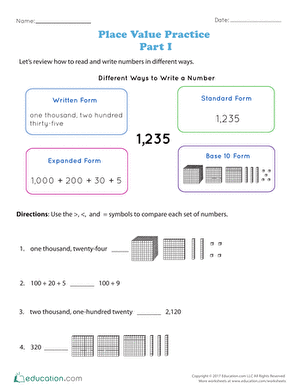Place Value Practice: Part I
Worksheet
Place Value Practice: Part I
Kids compare numbers written in different ways with this worksheet.
Math
Worksheet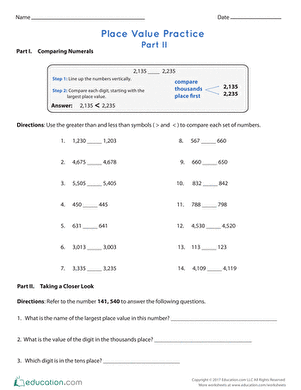Place Value Practice: Part II
Worksheet
Place Value Practice: Part II
Kids compare numbers in this place value worksheet.
Math
Worksheet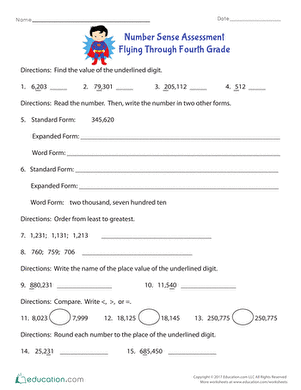Number Sense Assessment: Flying Through Fourth Grade
Worksheet
Number Sense Assessment: Flying Through Fourth Grade
Your students will apply their understanding of place value and number sense as they complete this math problem set.
Math
Worksheet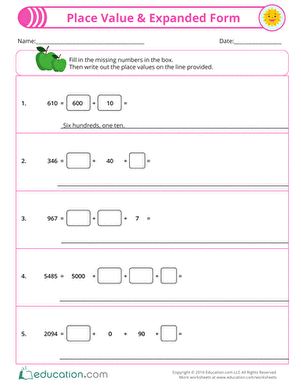Place Value & Expanded Form
Worksheet
Place Value & Expanded Form
Multi-digit numbers might be intimidating to a struggling math student. Help students practice breaking down big numbers by using place value with this worksheet.
Math
Worksheet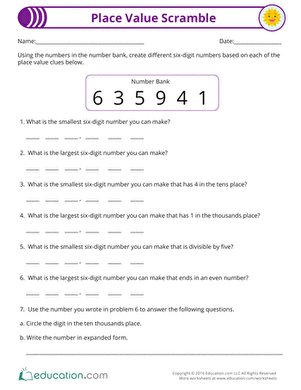Place Value Scramble
Worksheet
Place Value Scramble
Rise and shine, it's time for a math scramble! Students will apply their place value and number sense knowledge to create numbers that match the descriptions.
Math
Worksheet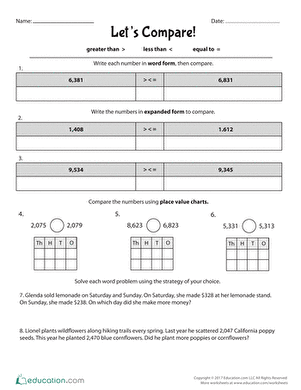Let's Compare
Worksheet
Let's Compare
This activity encourages students to compare whole numbers by using three different strategies. Students will break down numbers in written and place value form, then compare using symbols.
Math
Worksheet

## Lesson PlansGrowing by Powers of Ten
Lesson plan
Growing by Powers of Ten
Make multiplying by powers of ten more accessible to your students by focusing on patterns in the number of zeros!
Math
Lesson planLet's Compare Whole Numbers
Lesson plan
Let's Compare Whole Numbers
Equip your students with three great strategies to analyze place value and compare whole numbers up to the thousands place.
Math
Lesson plan

## Workbooks

No workbooks found for this common core node.

## Games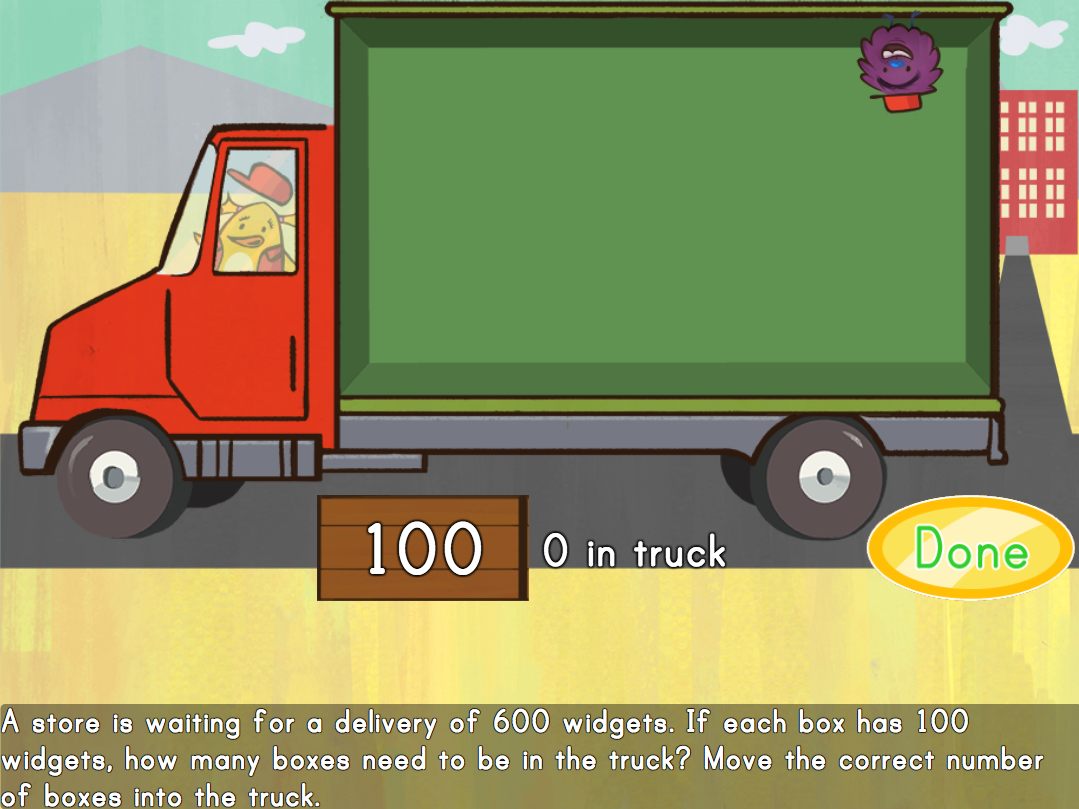Multiplying by 100s: Moving Truck Multiplication
Game
Multiplying by 100s: Moving Truck Multiplication
Kids help Birdee move cargo and solve multiplication by 100 in this manipulatives math game.
Math
Game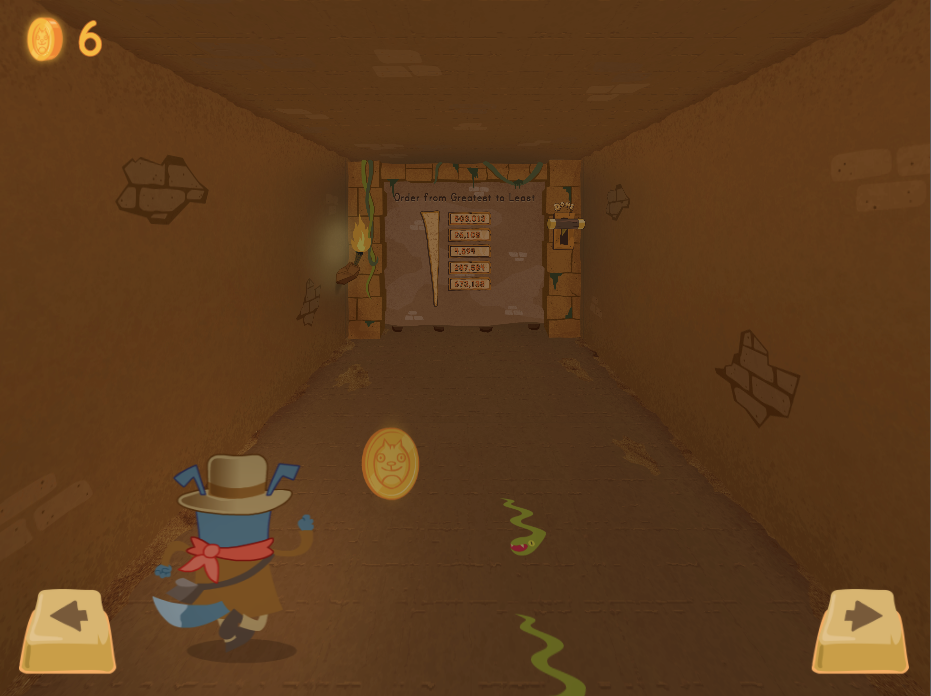Floyd Danger: Comparing Multi-Digit Numbers
Game
Floyd Danger: Comparing Multi-Digit Numbers
Help kids get through a mysterious tunnel in this engaging multi-digit number comparison game!
Math
Game

## Exercises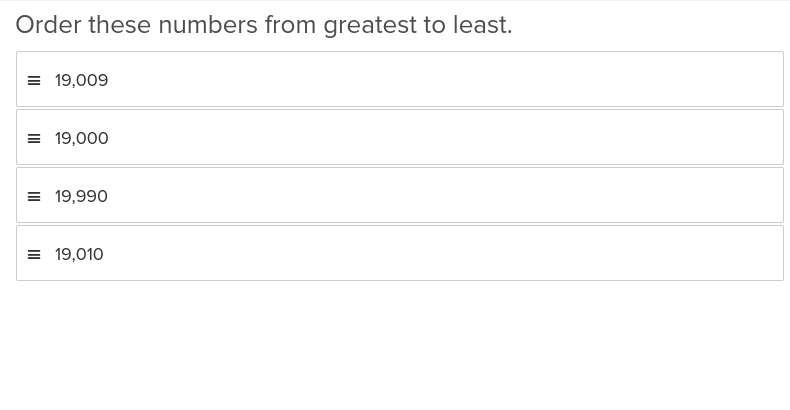Comparing Multi-Digit Numbers
Exercise
Comparing Multi-Digit Numbers
This elucidating Education.com exercise will show students working with multiplication, division, addition, or subtraction how to compare multi digit numbers.
Math
Exercise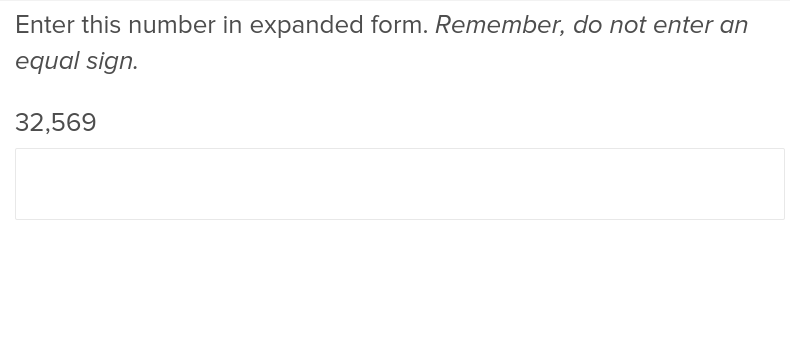Place Value and Numbers in Expanded Form
Exercise
Place Value and Numbers in Expanded Form
This exercise demonstrates how addition in larger numbers is simpler when numbers are dealt with in their expanded form.
Math
Exercise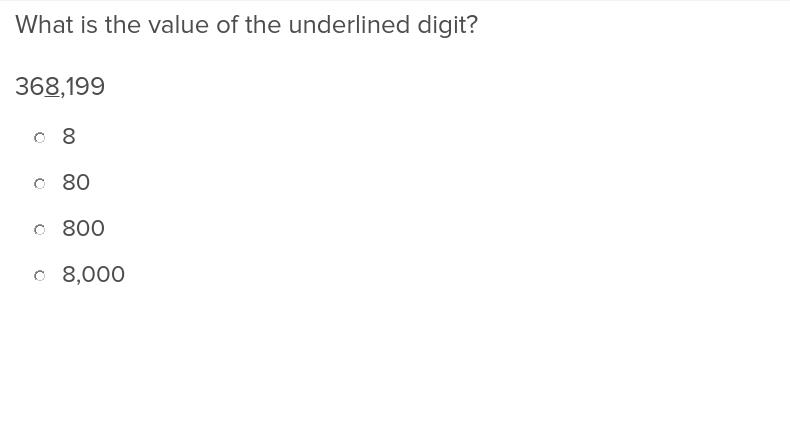Place Value Up to Ten Thousands Place
Exercise
Place Value Up to Ten Thousands Place
Build students’ confidence in solving math problems with large numbers using this exercise on place values up to the ten thousands place.